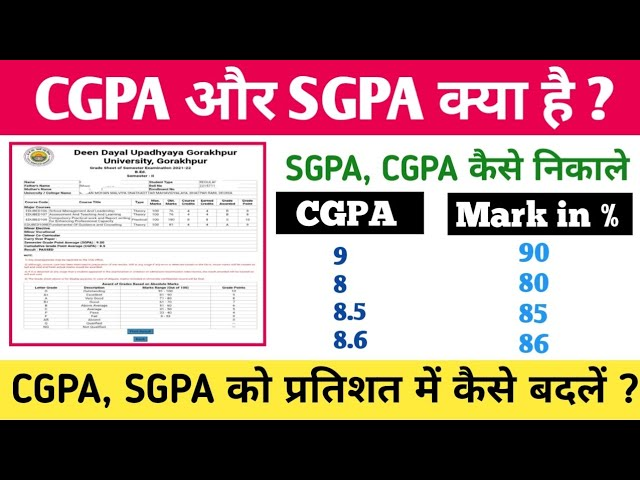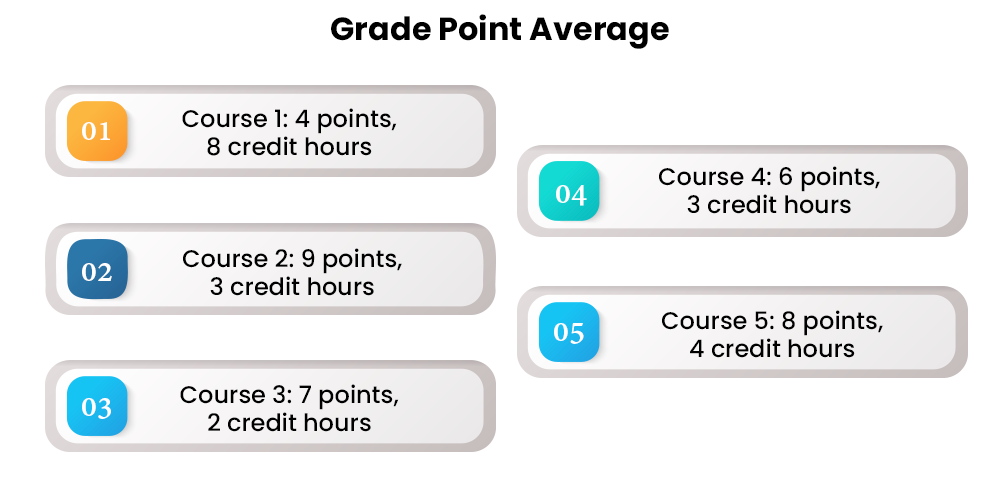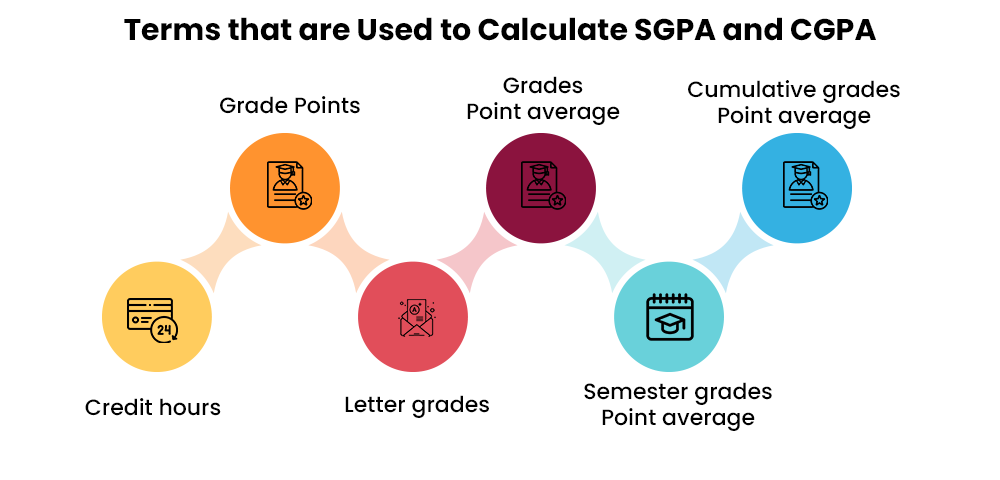Home CV Talks SGPA and CGPA: What it Is, How to Calculate, Difference

# SGPA and CGPA: What it Is, How to Calculate, Difference

## SGPA and CGPA: What it Is, How to Calculate, Difference

The grading system is essential to the academic world since it evaluates students' academic achievement. SGPA (Semester Grade Point Average) and CGPA (Cumulative Grade Point Average) are regularly used grading systems at colleges and universities worldwide. SGPA and CGPA are determined using the students' marks in their courses.

The SGPA evaluates a student's academic achievement in a given semester. It is computed by dividing the total number of credits taken that semester by the total number of grade points. The CGPA shows a student's academic performance throughout their studies.

Knowing the distinctions between SGPA and CGPA is critical for students in correctly determining their academic progress. For example, CGPA evaluates a student's overall academic performance for graduation or other academic requirements. In contrast, SGPA assesses a student's eligibility for scholarships and other educational incentives in a particular semester.

 You May Interested In Online Courses Global MBA Online Online MBA Courses MBA for Working Professionals MBA Distance Education MCA Online Courses BCA Courses Online Online MA Courses Online BA Courses

This article will go through SGPA and CGPA in-depth, including how to calculate them, their differences, and their importance in the academic world. In addition, students will thoroughly grasp various grading systems and how to utilize them to track their academic progress.Video Source: Mister Classes

## What is SGPA (Semester Grade Point Average)?

Semester Grade Point Average is abbreviated as SGPA. It is a grading system used in many colleges and institutions to assess a student's academic achievement for a given semester. The SGPA is determined using students' grades from all courses taken in a semester.

The semester grade point average determines a student's eligibility for scholarships, prizes, and other academic honors. It also gives students feedback on their academic achievement and assists them in identifying areas where they need to improve.

## How is SGPA calculated?

The student's SGPA is derived by allocating grade points to each grade received in their courses. The grade points are calculated by multiplying the letter grade's grade point value by the number of credit hours for that course. The SGPA is then determined by dividing the total number of credit hours attempted by the sum of the grade points received.

For example,

• If a student receives an 'A' in a three-credit hour course, the grade point value for an 'A' is 4.0, and the course grade point is 12. (4.0 x 3).
• If a student receives a 'B' in a two-credit hour course, the grade point value for 'B' is 3.0, and the course grade point is 6. (3.0 x 2).
• If a student receives an 'A' in a four-credit hour course, the 'A' grade point value is 4.0, and the grade point gained for that course is 16. (4.0 x 4).
• If a student receives a 'C' in a one-credit hour course, the grade point value for 'C' is 2.0, and the course grade point is 2. (2.0 x 1).

The student's overall grade point total for the semester would be 36 (12+6+16+2), and the total credit hours attempted would be ten (3+2+4+1). As a result, the semester SGPA would be 3.6 (36/10).## What exactly is CGPA?

CGPA is an abbreviation for "Cumulative Grade Point Average." It is a metric that measures a student's academic achievement over a certain period, generally an academic term or year, and is calculated on a scale of 0 to 4.0. The CGPA considers the student's grades in all courses taken during that period and provides each grade with a numerical value ranging from 0 to 4.0. The cumulative grade point average (CGPA) is computed by dividing the total number of grade points achieved by the total number of credits attempted. Schools and universities frequently use it for grading and entrance reasons to measure a student's overall academic achievement.

## How is CGPA calculated?

The cumulative grade point average (CGPA) is derived by taking the weighted average of a student's grade points from all semesters of their course. The CGPA formula is as follows:

CGPA = (Total Grade Points Achieved Throughout All Semesters) / (Total Credits Taken in All Semesters)

To compute the CGPA, first, total the grade points earned by the student in each semester. To do so, multiply the grade point allocated to each grade by the number of credits assigned to that course.

For example,

if a student receives an 'A' in a three-credit course, the grade point total for that course is

9 (A grade point) x 3 (course credits) = 27 grade points

Once you've computed the grade points for each semester, add them to get the student's overall grade point average for all semesters. Then, sum the credits earned by the student over all semesters.

Lastly, divide the total number of grade points earned by the total number of credits earned throughout all semesters. The resultant figure indicates the student's CGPA.

## In Demand Online Courses Specialization

 MBA Online In Banking and Finance MBA Online In Finance MBA Online In Marketing MBA Online In HR MCA Online In Computer Science and IT MSc Online In Mathematics MCom Online In Finance and Accounting BBA Online In Business Analytics MA Online In English BA Online In English

## How is CGPA different from SGPA?

The primary distinction between SGPA and CGPA is the measurement's breadth. For example, the Semester Grade Point Average (SGPA) measures students' academic achievement in one semester. In contrast, the Cumulative Grade Point Average (CGPA) measures a student's academic performance over numerous semesters or years.

SGPA is computed by allocating grade points to each grade received by the student in each course over a given semester and then averaging the grade points gained across all courses taken during that semester. CGPA, on the other hand, is computed by giving grade points to each grade received by the student throughout numerous semesters or years and then averaging the grade points gained across all courses.

In other words, SGPA is a snapshot of a student's academic success during a particular semester. Still, CGPA is a complete evaluation of a student's academic performance throughout their career. Universities and colleges frequently employ CGPA to assess eligibility for graduation, scholarships, and other academic honors, whereas SGPA tracks students' progress from semester to semester.

## How is CGPA calculated from SGPA?

To compute CGPA from SGPA, perform the following steps:

• Determine the total number of credit hours attempted throughout all semesters.

For instance, if a student tried 120 credit hours over eight semesters, the total number of credit hours attempted is 120.

• Calculate the total amount of grade points obtained throughout all semesters. Then, multiply the SGPA attained each semester by the number of credit hours tried to achieve this.

For example, if a student obtained an SGPA of 3.5 while taking 15 credit hours in the first semester, the total grade points gained would be 52.5. (3.5 x 15). Rep to this procedure for each semester.

• Total the number of grade points achieved over all semesters.
• Divide the total number of credit hours attempted by the total number of grade points received. The CGPA is the outcome of this calculation.

A student obtained an SGPA of 3.2, 3.5, 3.7, 3.9, 3.8, 3.6, 3.5, and 3.9 in eight semesters, taking credit hours of 15, 16, 14, 17, 18, 19, 16, and 15.

Each semester, the total number of grade points gained would be 48, 56, 51.8, 66.3, 68.4, 56, and 58.5.

The overall number of grade points gained across all semesters is 423.4 (48 + 56 + 51.8 + 66.3 + 68.4 + 56 + 58.5), and the total number of credit hours attempted is 120 (15 + 16 + 14 + 17 + 18 + 19 + 16 + 15).

When the total number of grade points obtained is divided by the total number of credit hours attempted, the CGPA is 3.53 (423.4/120).

## How do you convert a CGPA to a percentage?

The CGPA (Cumulative Grade Point Average) measures a student's overall academic achievement that is computed by averaging the grade points earned in each semester of a course.

Nonetheless, converting CGPA to a percentage is occasionally essential for numerous academic and career needs.

To convert CGPA to a percentage, use the formula:

Percentage = CGPA multiplied by 9.5.

The calculation is based on the fact that the highest grade point (10) equals 100% in the percentage system. To convert CGPA to percentage, multiply it by 9.5, the ratio of the highest grade point (10) to the highest percentage (100%).

For example,

suppose you have a CGPA of 8.2. You may use the following formula to convert it to a percentage:

77.9% = 8.2 x 9.5 = 77.9%

As a result, your CGPA of 8.2 is comparable to 77.9% in percentage terms.

It is crucial to note that the conversion formula may differ based on the institution's grading system. For example, to convert CGPA to a percentage, certain schools may employ a different maximum grade point or a different ratio. Therefore, to guarantee appropriate conversion, it is always advisable to check with the institution's requirements or confer with the relevant authority is always advisable.

## How to compute SGPA as a percentage:

To compute SGPA (Semester Grade Point Average) in percentage, divide the grade points earned in a semester and the total credit hours of courses completed that semester. The obtained SGPA may be converted to a percentage using the following formula:

• SGPA × Maximum Grade Point x 10 = Percentage.

The maximum grade point may differ based on the institution's grading system. For example, most institutions in India employ a 10-point grading system, with a top grade point of 10. As a result, the formula for calculating SGPA in % in India is:

• Overall Grade Points Acquired / Total Credit Hours = SGPA

Percentage = SGPA multiplied by 10 and multiplied by 100.For example,

suppose a student took five classes in a semester and received the following grades:

The total number of grade points received is (8x4) + (9x3) + (7x2) + (6x3) + (8x4) = 103.

The total number of credit hours would be 4+3+2+3+4=16.

As a result, the SGPA may be computed as follows:

• SGPA = 103/16 = 6.44 (rounded to two decimal places) (rounded to two decimal places)

The SGPA's percentage equivalent may then be computed as follows:

• 6.44 x 10 x 100 = 64.4%

As a result, the student's SGPA of 6.44 is comparable to 64.4% in percentage terms.

It is crucial to note that the conversion formula may differ based on the institution's grading system. Therefore, checking the institution's rules or conferring with the relevant authority is suggested to guarantee appropriate conversion.

The grading system used to assign grade points differs per institution. Most universities in India use a 10-point grading system. The followings are the grade points and their related grades:

 Grade Point Grade 10 A+ 9 A 8 B+ 7 B 6 C 5 D 0 F (Fail)

The grades 'A+,' 'A,' and 'B+' are regarded as good, whereas 'B,' 'C,' and 'P' are deemed mediocre. A grade of 'F' indicates that the student failed the subject.

The SGPA and CGPA are essential factors in determining a student's academic achievement. They aid in the evaluation of a student's development, the identification of strengths and deficiencies, and the implementation of essential modifications. Admission to higher education courses, job applications, and other academic endeavors require SGPA and CGPA.## Top Trending Articles

 Top 10 Online Degree Courses In India Business Communication: What It Is, Types and Importance Top 20 MS Excel Formulas and Function Bachelor of Commerce (B.Com): Types, Courses, Subjects 2023 How to Write a Good Article Industrial Safety Course Online (Diploma, Fees, Eligibility) Project Management Explained

## Conclusion

To summarise, the SGPA is essential for students to evaluate their academic performance, set objectives, track progress, and identify strengths and shortcomings. It assists pupils in identifying areas for growth, working on their weak points, and achieving the desired results. SGPA is also essential in determining employment options, making it an important indicator for students seeking success outside the classroom.

Remembering that SGPA should not be used as the sole indicator of academic performance is critical. Students should strive for comprehensive learning and practical knowledge beyond textbook study. Students should participate in co-curricular activities, internships, and real-world experiences that will allow them to develop soft skills such as teamwork, leadership, communication, and critical thinking.

Finally, SGPA is a crucial instrument that provides students with helpful information about their academic achievement. Students can attain intellectual brilliance and prepare for successful jobs by exploiting its benefits and concentrating on holistic learning.

The SGPA and CGPA grading systems assess a student's academic achievement. In addition, educational institutions utilize them for various objectives, including entrance, scholarship, and career prospects.

The SGPA is determined by summing the grade points received in all courses taken during a semester and dividing the total number of credits tried by the total number of credits attempted.

The CGPA is determined by summing the grade points received in all courses taken during an academic year or multiple years and dividing the total number of credits tried by the total number of credits attempted.

The SGPA measures a student's performance during a specific semester, whereas the CGPA measures a student's overall performance across an academic year or many years. In addition, the semester grade point average (SGPA) is computed at the end of each semester. In contrast, the cumulative grade point average (CGPA) is calculated at the end of each academic year or multiple years.

SGPA and CGPA grading scales differ based on the educational institution. Most schools, however, utilize a scale of 0 to 10, with 10 being the highest grade point.

The SGPA and CGPA are essential indicators of a student's academic achievement. They aid in the assessment of a student's strengths and shortcomings, the identification of areas for progress, and the provision of feedback to the student. They also decide whether or not a student is eligible for numerous academic programs, scholarships, and career prospects.

## Recommended for you

Tired of dealing with call centers!

Get a professional advisor for Career!

Rs.1499(Exclusive offer for today)Pooja

MBA 7 yrs expSarthak

M.Com 4 yrs expKapil Gupta

MCA 5 yrs exp

orHomeSearchSuggestUniversityStudentStoriesVideoCounseling